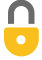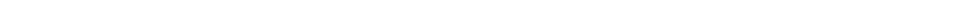Problem 64
A
$KE = 5 \times 0^{16} \textrm{J}$
$L = 3 \times 10^{20} \textrm{kgm}^2\textrm{/s}$ Note: At 3:23 I mention correctly that the angular velocity is $3.333 \times 10^{-4}$, whereas at 3:46 I incorrectly wrote $3.333 \times 10^{-11}$. My calculations were nevertheless done using $3.333 \times 10^{-4}$, so the results are correct. Where you hear $3.333 \times 10^{-11}$, please think: $3.333 \times 10^{-4}$.In order to watch this solution you need to have a subscription.By thesouthportschool on Sat, 2/18/2017 - 11:37 PM

Is the answer not 453.6926 J or 5*10^2 J, I copied the exact calculations from your final answer of part A (3:49) and did not get 5*10^16 J.

By thesouthportschool on Sat, 2/18/2017 - 11:43 PM

And I also you changed the angular velocity from 3.333*10^-4 to 3.333*10^-11 when doing the calculations to work out the KE

By Mr. Dychko on Wed, 2/22/2017 - 8:37 AM

Hello student from thesoutportschool, thank you for spotting the error. At 3:23 I mention correctly that the angular velocity is $3.333 \times 10^{-4}$, whereas at 3:46 I incorrectly wrote $3.333 \times 10^{-11}$. My calculations were nevertheless done using $3.333 \times 10^{-4}$, so the results are correct. I'll put a note above the video to use $3.333 \times 10^{-4}$ for the angular velocity.

All the best,
Mr. Dychko

Giancoli Answers, including solutions and videos, is copyright © 2009-2023 Shaun Dychko, Vancouver, BC, Canada. Giancoli Answers is not affiliated with the textbook publisher. Book covers, titles, and author names appear for reference purposes only and are the property of their respective owners. Giancoli Answers is your best source for the 7th and 6th edition Giancoli physics solutions.